Reach Us+44-1522-440391
Noise Tolerance Analysis for Reliable Analog and Digital Computation in Living Cells | OMICS International
Journal of Bioengineering & Biomedical Science

Like us on:

All submissions of the EM system will be redirected to Online Manuscript Submission System. Authors are requested to submit articles directly to Online Manuscript Submission System of respective journal.

# Noise Tolerance Analysis for Reliable Analog and Digital Computation in Living Cells

Rizik L, Ram Y and Danial R*

Biomedical Engineering Department, Technion – Israel Institute of Technology, Haifa, Israel

*Corresponding Author:
Danial R
Biomedical Engineering Department
Technion– Israel Institute of Technology, Haifa, Israel
Tel: 972 77-887-5555
E-mail: [email protected]

Received: April 05, 2016; Accepted: April 20, 2016; Published: April 27, 2016

Citation: Rizik L, Ram Y, Danial R (2016) Noise Tolerance Analysis for Reliable Analog and Digital Computation in Living Cells. J Bioengineer & Biomedical Sci 6:186. doi:10.4172/2155-9538.1000186

Copyright: © 2016 Rizik L, et al. This is an open-access article distributed under the terms of the Creative Commons Attribution License, which permits unrestricted use, distribution, and reproduction in any medium, provided the original author and source are credited.

Visit for more related articles at Journal of Bioengineering & Biomedical Science

#### Abstract

Biomolecular computing, encompassing computations performed by molecules, proteins and DNA, is a central area of focus in Synthetic Biology research and development, which attempt to apply engineering design principles in living cells. Two major computation paradigms have been implemented so far in living cells - analog paradigm that computes with a continuous set of numbers and digital paradigm that computes with two-discreet set of numbers. Here, we analyze the biophysical and technological limits of large-scale gene networks created based on analog and digital computation in living cells. More specifically, we calculate the precision of analog systems and the noise margin of digital systems in living cells. We conclude that both systems are challenging to operate with low protein levels. To overcome this challenge, we show that analog systems should operate with a Hill coefficient smaller than 1 and digital systems should be buffered. Furthermore, an analytical description of a biophysical model recently developed for positive feedback linearization circuits and used in analog synthetic biology, is presented. Finally, we suggest new directions for engineering biological circuits capable of computation.

#### Keywords

Analog computation; Digital computation; Synthetic biology; System biology; Stochastic models; Control systems

#### Introduction

Computation has become an integral part of our evolution and marks a significant landmark in modern technological revolutions. The first abacus “calculator” was invented before 2000 BC and was based on counting continuous numbers, a process known today as an analog computation. However, scaling the complexity of computation was only truly achieved in the last century, when the digital transistor that counts discrete values, was invented. Computation based on digital design is relatively straightforward, with clear ON and OFF states that can and provide reliable results and form the basis for screening. Furthermore, digital circuits, with tightly controlled physical parameters, can be simply assembled to form complex networks, with very low cross-talk between components. The evolution of digital computation mainly relies on shrinking the transistor dimensions, which have almost reached the fundamental physical limits of scaling laws, breaking Moore's law. In contrast to digital design, analog design computes with a continuous set of numbers, with each wire carrying many bits of information. In addition, it uses the powerful laws of physics, that are naturally embodied in analog transistors, to execute sophisticated computational functions (e.g. addition, subtraction, multiplication, division, logarithms and power laws). The evolution of analog computation mainly relies on feedback loops to improve precision, attenuate noise and expand the working dynamic range .

The last decade witnessed major breakthroughs in biophysics and genomic technologies. Researchers have successfully applied biophysical models, by combining several genes to create basic biological networks with predictable behaviors in living cells [2-4]. At the same time, thanks to nanotechnology and biotechnology, significant advancements in genome DNA engineering and assembly techniques have been achieved . An outcome of these advancements is an extraordinary set of design rules and engineering tools that enable massive reprogramming of the DNA code in living organisms, including humans. This new technology, known as "synthetic biology" [6-8], attempts to translate engineering design principles to rational biological design [9,10], to achieve multi-signal integration and processing in living cells for diagnostic, therapeutic and biotechnological applications [11-14]. For example, living cells can be programmed to produce pharmaceutical compounds that are extremely challenging to synthesize using existing methods , microbiome bacteria can be programmed to detect and respond to changes in clinical homeostatis , and gene circuits can be engineered to identify and eliminate cancer cells . These developments constitute a milestone that marks the beginning of new biomolecular computing technologies, based on nano scale-level genecircuits in living cells that set an alternative limit to Moore's law.

Early efforts at biomolecular computing have used binding and unbinding reactions to represent the "ON/OFF" or "1/0" logic states. Consequently, proteins that bind to DNA or promoters and activate high levels of gene expression, represent the"1" logic state, while unbound, free proteins yield low levels of gene expression, andrepresentthe"0" logic state. Many genetic circuits that mimic electronic digital circuits, have been constructed to perform Boolean logic gates [16-18], counter  and memory  devices in living cells. However, because signals in living cells are graded in their nature [21-24] and do not generally exist in only two possible states, digital paradigms are often an oversimplified means of describing signals in living cells. Thus, such representation can lead to errors in construction and implementation of genetic circuits and challenge gene-network scaling in living cells [22,23].

To date, engineered artificial logic gates in living cells have been proven difficult to scaledue to cellular resource limitations, a lack of orthogonal genetic devices, high leakage levels of synthetic genetic devices and the absence of suitably sharp input-to-output transfer functions [22,23]. Recently, genetic circuits have been constructed based on analog design . Such gene circuits take advantage of the complex operations already naturally present in living cells, to execute sophisticated computational functions. For example, analog genetic circuits exploit positive feedback loops to implement logarithmically linear sensing, addition, division  and negative feedback loops while performing square-root calculations to determine chemical concentrations . Analog genetic circuits involve fewer components and resources, and execute more complex operations than their digital counterparts [21,24,25]. For example, an analog adder can be achieved by simply combining two parallel circuits, where each accepts different input molecules and produces common output molecules . This lies in sharp contrast to digital adders, which sum two “1” binary numbers, and require another stage to hold the new bit “Carry out” (“10”). For instance, a 4-bit digital adder may require more than 30 synthetic parts to operate, and at the same time, would place a substantial metabolic burden on a cell . By analogy to electronics, noise in biological systems [26,27] can set the physical and technological limits of engineered analog-design large-scale gene networks based in living cells. For an in-depth analysis of the pros and cons of analog versus digital computation in living cells and electronics, readers are referred to excellent reviews on the subject [1,25].

In the present article, we analyze the biophysical and technological limits of large-scale gene networks created based on analog and digital computation in living cells. The working dynamic range, noise margin, basal (leakage) level of biological parts, sharpness of input-to-output transfer functions and copy number of synthesized proteins/molecules are assessed. In the second part of this paper, we analyze analog computation in living cells. We close the work with suggestions for future directions for engineering computation functions in living cells.

#### Accuracy of Analog Systems in Living Cells

Figure 1a shows two computational elements in living cells; in the first one, the biochemical reaction occurs at the protein-DNA level. It includes an input protein signal (x) that binds to a promoter and activates transcriptional and translational processes to synthesize an output protein signal (z). In the second element, the biochemical reaction occurs at the chemical/protein-protein level. Both biocomputing elements can be described by a Michael is–Menten enzyme– substrate binding reaction via a Hill function, given by: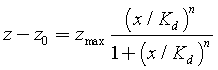(1)

Figure 1: (a) Basic bio-computing elements in living cells, including a protein that binds to a promoter and an inducer that binds a promoter (k1 is the forward rate of the binding reaction, k-1 is the reverse rate of the binding (unbinding) reaction) (b) Analog mode: input-to-output transfer function of equation 1 (blue line) and log-linear function at y=0 (black line) (c) Digital mode: Equation 1 represents two logic states "0/1" (d) Noise analysis of log-linear analog systems, (e) Total noise is the sum of amplified extrinsic noise and intrinsic noise in a log-linearanalog systems.

where, Kd is a dissociation constant of a biochemical reaction (Kd=K-1/K1), z0 is the basal level of binding, zmax is the maximum protein concentration achieved by the system, and n is the Hill coefficient, describing cooperativity. Figures 1b and 1c describe the input-to-output transfer function of Equation 1, which includes two regions: an analog continuous mode and a digital mode. In the analog mode, the function can be described by a log-linear transduction (z vs y =log(x / Kd ) , while in the digital mode, it can be viewed as two discreet values ("0" and "1"). Equation 1 can be approximated at x=Kd or (y=0), using Taylor series, as: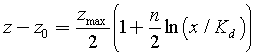(2)

Log-linear transduction, known as Weber's Law, is widely used in natural systems, such as audition, vision and cells , and offers advantages over linear-linear transduction. For example, small changes in the output of log-linear systemsare proportional to small changes in the input signals divided by their intensity (Δz ∝ Δx/x), demonstrating a memory element in the system. In contrast linear-linear systems show proportionality between small changes in the output and small changes in the input signals only (Δz∝Δx). The input dynamic range (IDR) in an analog mode is defined as (Figure 1b):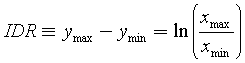(3)

Where z(x = xmax )-z0 =0.8a and z(x = xmin)-z0 =0.2a . Under there definitions, the error between the log-linear analog function (Eq. 2) and a Hill function (Eq. 1) at the limits of the IDR, is less than 5%. By substituting xmax and xmin in equation 3, IDR is then given by: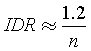(4)

Equation 4 shows that by decreasing the Hill coefficient or the sharpness of the input-output transfer function of the binding reaction, one can increase the log-linear range. In natural biological systems, the Hill coefficient typically ranges between 1 and 4 , and then the IDR varies between 1 to 0.25 orders of magnitude. Recently, Danial et al. showed that by implementing a graded positive feedback loop in synthetic biological systems, one can increase the IDR by 4 orders of magnitudes .

Signals often originate from the transport of discrete random carriers in systems; in electronics, it is a drift/diffusion of electrons , in physics, it is the movement of photons and in biology, it is the diffusion of biochemical molecules and proteins [27,29]. Naturally, these signals propagate through networks with random fluctuations, which can be described by a Poisson process, generating shot noise that scales as the square-root of the molecular count . Here, we analyze the design rules, determined by laws of cellular noise, which set the performance limits of analog and digital biological systems. Typically, there are two orthogonal sources of noise in any biological system [29,30]. The first source is the intrinsic noise, generated by the system itself, and the second source is the extrinsic noise, generated by random fluctuations in the input or another environmental parameter. A stochastic model for cellular intrinsic noise may be greater than Poisson process, with addition of burst size (bint) is given by :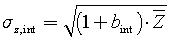(5)

The burst size in a gene expression model is the average number of proteins synthesized (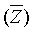) per mRNA transcript. In a simple enzyme– substrate binding reaction, the cellular intrinsic noise is given by a Poisson process only. For simplicity, we assume that the system is operated at x=Kd and then, if z=zmax/2 is substituted in equation 5, we get: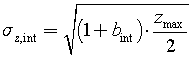(6)

The gain of an analog system in a log-linear mode, amplifies random fluctuations in the input signal (Figure 1d). Then, the contribution of extrinsic noise (σy) on the output signal at x=Kd in a log-linear mode is expressed by: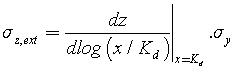(7)

The input y, is described by a log-linear function with input x, and therefore, the noise of y at x=Kd is a function of the noise of x (σx), and is given by: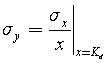(8)

The input x, is a number of proteins or chemical molecules and thus, its noise (σx) can be described by a Poisson process, with addition of burst size (bext)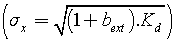. By substituting the last term of σx in Equation 8, we get: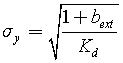(9)

Equations 8 and 9 reveal that the noise in log-linear systems scales as the inverse of the square root of the molecular count, in contrast to linear-linear systems, where the noise scales as the square-root of the molecular count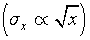. It is simple to show that the gain of a loglinear system at y=0 (or x=Kd) is equal to: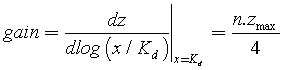(10)

By substituting Equations 9 and 10 into Equation 7, we find that the contribution of the extrinsic input noise on the output signal is: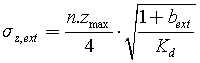(11)

Because the intrinsic and extrinsic noise or thogonally contribute to the total noise of the system (σz) , the total noise can be given by: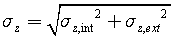(12)

If we substitute the values of intrinsic and extrinsic noise, i.e., Equations 6 and 11, respectively, into the last formula, we find that the total noise in the output of the analog signal in biochemical reactions is given by: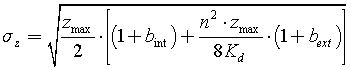(13)

Any small change in the input (Δy), within the IDR range, is amplified by the gain of the system and yields a change in the output (Δz=gainÎ�Δy, Figure 1d and 1e). Biological systems have a log-linear transduction and therefore, the change of the output (Δz) as a response to change in the input (Δy=Δx/x) at x=Kd, is given by: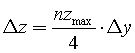(14)

Forimproved performance of analog systems, we require that changes in output are larger than the total noise of the system (Δz>σz) (Figure 1d). Thus, the minimum change in the input (Δymin) is given by: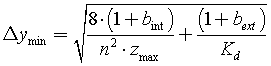(15)

Equation 15 suggests that increasing the Hill coefficient (n), or the sharpness of input-to-output transfer function, of analog biological systems improves their performance. However, as we have shown in Equation 4, the IDR is reduced for high values of n, thereby affecting system performance (e.g., for a high value of n, the IDR can be smaller than the minimum change in the input (Δymin), thereby reducing the system’s performance). Therefore, we define the precision of an analog system, which is equivalent to the signal-to-noise ratio, as the number of levels that the system can distinguish in the presence of noise. This can be calculated as the ratio of IDR (Equation 4) and minimum changes in the input (Equation 15):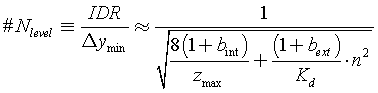(16)

Equation 16 represents the precision of analog systems in a loglinear mode, when we consider the contribution of extrinsic/intrinsic noise and the input dynamic range. The equation suggests that for high molecular counts or protein copies, the precision of the system will be enhanced. It also shows the contribution of extrinsic noise, which depends on a Hill coefficient, and the contribution of intrinsic noise, which is independent of a Hill coefficient. We now analyze Equation 16 under two different conditions, in accordance with the proposed basic bio-computing elements in living cells (Figure 1a). In a chemical/ protein-protein reaction, the dissociation constant is often larger than the maximum protein copy number (zmax<<Kd), and therefore, Equation 16 can be approximated as: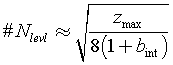(17)

In this case, the intrinsic noise can be viewed as the fluctuations in chemical/protein-protein binding and protein synthesis. While Equation 17 is only an approximation, it describes the precision of analog systems when the extrinsic noise is small. Under these conditions, the precision of the system is set only by the maximum protein copy number achieved by the system and by the intrinsic noise (Figure 2a), independent of IDR.

Figure 2: Noise Tolerance Analysis for reliable analog computation in living cells.
(a) The figure shows the precision of analog systems in a log-linear mode, when we take into account the contribution of intrinsic noise and input dynamic range (Equation 17) (b) The figure shows the precision of analog systems in a log-linear mode, when we take into account the contribution of extrinsic/intrinsic noise and input dynamic range (Equation 18).

Analog systems can be alternative to their digital counterparts (1 bit of output precision) when operating with 4 to 8 levels of information (equivalent to 2-3 bits of output precision), which, based on our analysis (Figure 2a), can be achieved with 1000 proteins copies or molecular counts. In Escherichia coli, 1000 molecule counts is equal to a concentration of 1μM, which is typically the levels of signaling proteins  (e.g., it was found that there are roughly 100 copies of EnvZ per cell and around 3500 copies of OmpR).

Protein-DNA biochemical reactions that involve transcription and translation processes, often operate with low protein copy numbers . For such systems with both intrinsic and extrinsic noise sources, precision in a log-linear mode is described by Equation 16. For simplicity, we rearranged Equation 16 and assumed that the number of input and output protein copies are equal (Kd=Zmax/2) and the burst size for intrinsic and extrinsic noise is also equal (bint=bext=b):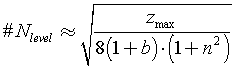(18)

The burst sizerelies on the translation rate, number of amino acids (aa) in the synthesized protein and on mRNA half time. Typically, in Escherichia coli, the translation rate ranges between 10-20aa/sec, depending on growth conditions , and mRNA half time is around 3-5 min . Therefore, the burst size in Escherichia coli, can range between 3-15. Figure 2b shows that, to achieve proper performance of analog systems based on protein-DNA biochemical reactions with 4-8 levels of information (2-3 bits of precision), the effective Hill coefficient should be smaller than one. The measured Hill coefficient in natural biological system is often higher than one, therefore, there are challenges in creating analog genetic circuits.

#### Analog Computation in Living Cells

The first step toward implementation of synthetic analog computation in living cells, is to broaden the input dynamic range of genetic synthetic parts. Protein-DNA interactions typically have a narrow dynamic range, spanning 0.5 - 1 orders of magnitude. The input dynamic range of genetic parts is set by the cooperative binding of proteins to DNA and is often positive, with a Hill coefficient larger than one. This would mean that once one protein is bound to a DNA binding site, its affinity for other proteins increases. By contrast, a negative cooperative binding reaction has a Hill coefficient smaller than 1. Dainal et al.  implemented a positive feedback loop and decoy binding sites to shunt the proteins away from their target binding site, and achieved a Hill coefficient smaller than 1, with a very wide input dynamic range. Comprehensive biophysical and biochemical reaction models that fit their experimental results were presented . In this article, we show a new analytical model that can explain the contribution of a shunt on an open loop and positive feedback loop. Figure 3a describes a transcription factor x(TF) that binds to m identical promoters. The m-1 binding reactions act as a decoy or shunt pathway for the transcription factors. For simplicity, we assume that the Hill coefficients for all the promoters are equal to 1. The biochemical reaction model of this system is presented in Figure 3b and its solution in steady state is given by: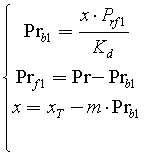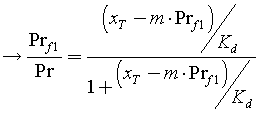(19)

Figure 3: (a) Open loop and shunt circuit: a transcription factor binds to m identical promoters, (b) biochemical reaction model of an open loop and shunt circuit, (c) a schematic model includes Michaelis–Menten and a negative feedback for the open loop and shunt circuit (d) Simulation results show the contribution of shunt biochemical reactions on the activity of the target promoter (e) The simulation results show the contribution of the shunt biochemical reactions on the effective dissociation constant.

where Pr is the total number of target promoters, Prf is the number of free target promoters, Prb is the number of target promoters occupied by transcription factors, xT is the total number of transcription factors and Kd is the dissociation constant of the binding reaction. Equation 19 can be viewed as a Michael is–Menten (MM) model (equation 1) with a negative feedback (Figure 3c). The addition of decoy or shunt pathways increases the strength of the negative feedback loop and shifts the switch point of input-output transfer function to higher values (Figure 3d). If we fit the simulation results of Equation 19 to a Hill function, we find that the effective dissociation constant scales with the number of shunt reactions (Figure 3e; Kdeff=mÎ�Kd). For a very large number of shunt reactions or very strong negative feedback loops, Equation 19 can be approximated as a linear-linear function (Figure 3d), with a very weak signal and is given by: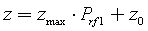(20.1)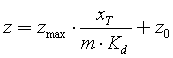(20.2)

Equation 20 represents the copy number of synthetized proteins in an open loop and shunt circuit. To amplify the weak signal of the open loop circuit, a positive feedback loop regulating only the target promoter, was included . Figure 4a shows the new positive feedback loop and shunt circuit. Danial et al. used external inducers (e.g., AHL as a quorum sensing molecule or arabinose) to trigger the positive feedback loop and shunt. A simple model of the circuit is presented in Figure 4b and includes three elements: (1) a linear circuit that demonstrates the contribution of shunt reactions (Equation 20), (2) a positive feedback loop, and (3) a multiplication operator. The inducertranscription factor binding reaction is modeled by a multiplication between the transcription factor and Hill function (xT=z*f(In)). By substituting the last expression into Equation 20, we can express the solution of a graded positive feedback loop and shunt circuit as: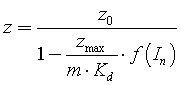(21)

Figure 4: (a) Positive feedback loop and shunt circuit: the transcription factor is produced by its own promoter and binds to m identical promoters (b) A schematic model of positive feedback and shunt circuit includes a linear part (c) Simulation and analytical results showing a graded positive feedback loop (d) A schematic model for the input dynamic range of positive feedback shows the contribution of shunt biochemical reactions.

We can distinguish between two cases: (1) a very strong (zmax/mÎ�Kd>>1) positive feedback loop, which yields a sharp input-output transfer function. In this case, the inducer-output protein transfer function is set by the transcription factor–promoter binding reaction and inducer-transcription factor binding reaction. The solution in this case is obtained by substituting xT=z*f(In) into Equation 19 (Figure 4c). (2) A graded positive feedback (zmax/mÎ�Kd<<1), which yields a loglinear transduction between input and output (Figure 4c). This can be achieved by increasing the number of shunted biochemical reactions, or by decreasing the binding efficiency of transcription factors to the promoter, or decreasing the translation/transcription rates of proteins affecting zmax. In this case, the inducer-output protein transfer function is set by the inducer-transcription factor binding reaction only and is given by: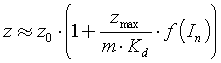(22)

Figure 4c shows that the reduction of zmax/mÎ�Kd broadens the input dynamic range of the positive feedback circuit. We can see that our analytical model fits (Equation 22) the exact model constructed based on biochemical reactions. The positive feedback loop and shunt circuit cannot widen the input dynamic range (IDR) more than the dynamic range of the inducer-transcription factor binding reaction (Figure 4d). The maximum signal that can be achieved in such a system is z=z0Î�(1+zmax/mÎ�Kd), and therefore, the addition of shunt biochemical reactions decreases the signal output. A simple explanation was provided by Danial et al. , who suggest that the shunt creates several binding sites that delay the saturation of the transcription factor-binding site reaction at the target promoter. At the same time, as the inducer concentration increases, the positive feedback loop enables continuous production of just enough transcription factors.

Synthetic analog parts that operate in a log-linear mode with a wide input dynamic range, can be simply integrated into more complex circuits for higher order functions . For example, a genetic analog adder has been constructed in living cells by simply combining two analog synthetic parts (e.g., positive feedback loop and shunt) that each accept different input molecules and produce the same output molecules . The addition operator was achieved by summing up the common diffusion fluxes of output molecules . This operation is equivalent to Kirchhoff's current law in electronics. By contrast, a genetic digital adder cannot be constructed using the same principle that exploits a common output signal, since every wire in digital design represents only a bit of information, and would require an additional stage to hold the carry out. For example, building a half 1 bit adder in bacteria requires7 synthetic parts [32-34]. Analog computation presents an alternative to digital computation when the number of synthetic parts is limited. An analog subtractor can be constructed using the same principles applied for the analog adder . The analog subtractor has two log-linear stages that produce common output proteins, one stage with a positive slope and another stage with a negative slope. Danial et al.  has used a LacI repressor to implement an analog stage with a negative slope.

#### Noise Margin of Digital Systems in Living Cells

Figure 1c describes the input-to-output transfer function of Equation 1 in a digital mode, as log-log(s =log(z / zmax )vsy =log(x / Kd)) . It can be viewed at two discretelevels (low and high):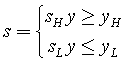(23)

This device demonstrates a buffer logic gate operating in its extreme regions. This is exactly the opposite of its use as an analog device, where it operates in a log-linear region, at the middle of the transfer function (x=Kd). Digital logic gates utilize the gross nonlinearity exhibited by biochemical reactions in living cells. With these observations, the lowlevel output (sL) does not depend on the exact value of the input signal (y) as long as it does not exceed the low-level input (yL). Similarly, we observe that high-level output (sH) does not depend on the exact value of the input signal (yL), as long as its value does not fall below the high-level input (yH). When the input signal is higher than the lowlevel input and lower than the high-level input (yL<y<yH), the output increases and the logic gate enters its transition region, where the device can only act as an analog device. Similarly, we can define the logic levels for others logic gates. Ideal digital logic gates have a zero width of transition region and infinite sharpness of input-to-output transfer functions (very high Hill coefficient), operating in the middle of their transfer function at x=Kd (yL=yH=0), with maximum gain. However, the presence of intrinsic and extrinsic noise in biological and electronic systems limits the performance of ideal logic gates and drives a transition region with an input noise margin (INM) and output noise margin (ONM):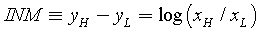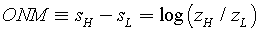(24)

The minimum INM will be set by the extrinsic noise of the input system and is given by: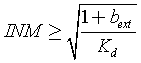(25)

In Equation 24, we assumed that the buffer logic gate operates at x=Kd (INM>σY). As we have shown, the transition region (or IDR in analog systems) is set by a Hill coefficient. For simplicity, we approximate the INM≈1/n (Equation 4). Then, Equation 25 can be given by: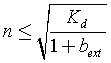(26)

Equation 26 is demonstrated in Figure 5a, which shows that for a low level of input protein (or a low dissociation constant; for simplicity we assumed that Kd=Zmax/2), the digital logic gate should operate with a very high input noise margin and a low Hill coefficient. Under these conditions, the system has a graded behavior, acting as an analog system. Our analysis has shown that noise limits the performance of both analog and digital systems in living cells, rendering them extremely challenging to operate with low level of proteins. Alternatively, operating with a high level of input proteins can improve the performance of digital systems and reduce the INM. However, it increases the output noise margin. To quantify this insensitivity property, we consider the situation that often occurs in digital systems, where one buffer logic gate drives another buffer logic gate (Figure 5b). In this case, the digital cascade can only operate properly when the lowlevel output (sL) of the first stage is lower than the input level (yL) of the second stage and when the high-level output (sH) of the first stage is higher than the input level (yH) of the second stage. The output of the first stage often includes an intrinsic noise which sets the limits on the performance of the cascade (Figure 5b), and therefore we can write: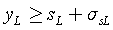(27.1)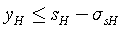(27.2)

Figure 5: (a) Input noise margin analysis for digital circuits in living cells (Equation 26) (b) Contribution of intrinsic noise to the output noise margin in digital circuits in living cells (c) Contribution of noise margin on cascading digital circuits in living cells (d) Output noise margin analysis for digital circuits in living cells (Equation 29).

Because the relation between the output s and output z is described by a log-linear function, the noise of output s is given by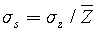and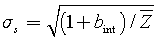Subtracting Equation 27.2 from Equation 27.1, i.e., substituting the noiseof the output sand assumed that zH>>zL gives: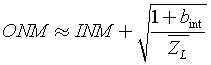(28)

To better understand Equation 28, we will cascade three identical buffer logic gates with zL=10 and bint=9 (Figure 5c). The resulting output noise margin of every layer i is larger than its input noise margin by one order of magnitude (ONMi=INMi+1), and the output noise margin of the last layer is larger than the input noise margin of the first layer by three orders of magnitudes (ONM3=INM1+3). For example, constructing a cascade of three logic layers, with an initial input noise margin of one order of magnitude, causes the last stage to have a very wide input dynamic range spanning 4 order of magnitudes (Figure 5c). Alternatively, we can increase the low-level output to 100 molecules, achieving ONM≈INM, however in this case, the high-level output is set to very high values. The basal level (z0) in synthetic biological parts, is often very large, and therefore, it sets the low-level output of digital systems (zL≈z0).If we substitute the INM in Equation 25 into Equation 28, we find other important relations: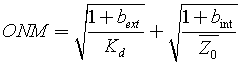(29.1)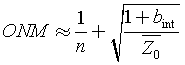(29.2)

The last two equations quantify how the output noise margin of digital systems in living cells relates to intrinsic and extrinsic noise sources, Hill coefficient, basal level and molecule counts. Based on our analysis, in contrast to analog systems, the basal level is extremely important in determining the performance of digital systems (Figure 5d).

Digital computation in living cells have been widely used in synthetic biology and have been reviewed in several articles [7,8,33]. In this article, we briefly reviewed and discussed two key synthetic digital devices that were implemented in living cells. In the AND logic gate, output is only high if all inputs are high. The devices were constructed in bacteria [34,18], yeast  and mammalian cells , using a binding reaction between two synthetic parts regulated by input promoters. For example, Nissim et al.  constructed a system with two inputs that are duplicates of endogenous promoters that regulate the expression of a two-hybrid system, with one part fused to an activation domain, and the other to a binding domain. Together, they form a transcriptional complex that can bind a synthetic output promoter to express an output gene. By design, output is only generated if both endogenous promoters are active in the cell above a specific threshold. In the OR logic gate, output is high if at least one input is high. This device was constructed using two promoters that regulate the same gene . Taking different approaches, several groups have constructed logic gates and memory using recombinase proteins .

#### Summary

Synthetic and Systems Biology have recently learned to exploit analog and digital genetic circuits for computation and decision making. In this work, we analyzed the precision of analog systems (Equation 18) and the noise margin of digital systems (Equation 29). We demonstrated that the performance of analog and digital systems in living cells is significantly impacted by extrinsic and intrinsic noise sources. We showed that both systems are challenging to operate with low protein levels and that both systems require optimization. For example, analog computation operates with Hill coefficients smaller than 1and cascading of digital systems increases the input noise margin, conditions under which the digital system has a graded behavior acting as an analog system. We also have shown that, in contrast to analog systems, the basal level is extremely important in determining the performance of digital systems. Furthermore, we argue that, compared to digital design, analog computation is very efficient in its use of synthetic parts, however, embedded digital systems can operate reliably with low molecular counts. Therefore, biological systems that integrate both analog and digital circuits may provide an alternative strategy for scaling the complexities of computation in living cells [24,25]. Although this design is widely used in electronics, in such contexts, it mostly aims to convert analog signals to a two-logic states and not to build efficient systems. Therefore, in our opinion, a hybrid analogdigital architecture in living cells should take a different approach than in electronics.

#### Funding

This study is supported by Neubauer Foundation

#### Competing Interests

The authors declare no competing or financial interests.

#### References

Select your language of interest to view the total content in your interested language

### Article Usage

• Total views: 8862
• [From(publication date):
May-2016 - Sep 21, 2019]
• Breakdown by view type
• HTML page views : 8701

## Post your commentCan't read the image? click here to refresh
###### Peer Reviewed Journals

Make the best use of Scientific Research and information from our 700 + peer reviewed, Open Access Journals

International Conferences 2019-20

Meet Inspiring Speakers and Experts at our 3000+ Global Annual Meetings

Top